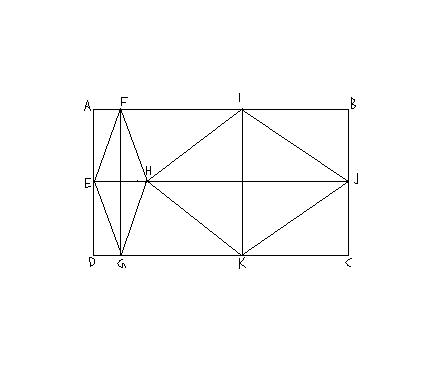SEARCH HOMEMath Central Quandaries & QueriesHi, my name is "malik". would u plz send me the answer of this question as soon as possible. my question is: ("rhombus" diagram is attached) ABCD and EJCD are rectangles. EFHG and HIJK are similar rhombus. HIJK is 4times bigger than EFHG. In each rhombus the larger diagonal is double of the smaller one. If AB = 30cm, what is the length of the diagonals of the smaller rhombus ?Malik,

When you say "HIJK is 4times bigger than EFHG." I expect that you mean the area of HIJK is 4 times the area of EFHG. Use this fact and the fact that the rhombi are similar to show that |HJ| = 2 |FG|. You also know that |FG| = 2 |EH| and hence

30 = |AB| = |EH| + |HJ| = |EH| + 2 |FG| = |EH| + 4 |EH| = 5 |EH|

PennyMath Central is supported by the University of Regina and The Pacific Institute for the Mathematical Sciences.